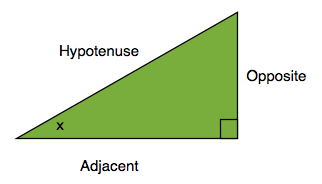# Trigonometry: Sine, Cosine, and TangentThis post is part of a series of posts on Trigonometry. To see all the posts, click on the tag #TrigonometryTutorials below. This is the post you should read before you read this.

In a Right-angled Triangle, take one of the angles that isn't the right angle and call it $x$. The side that is the longest and is opposite to the right angle is called the Hypotenuse, as you already know.

The side that is next to the angle $x$ and isn't the Hypotenuse is called the Adjacent side, a good way to remember this is that adjacent means "next to"

The remaining side is called the Opposite side, you can remember this because the side is opposite to the angle $x$

Notice if you chose the other angle that isn't $x$ the Adjacent and the Opposite sides would have switched around.

As I have said in my previous post, A Triangle is stable and if you know the 3 angles of a triangle you can find the ratio of the 3 sides of the Triangle. This is what the Trig ratios: Sine, Cosine, and Tangent are for.

Sine, Cosine and Tangent are often abbreviated to $\sin$, $\cos$, and $\tan$ and I will also do so when I refer to them later on.

This is what the Ratios are:

For a Right-Angled Triangle and an angle $x$:

$\sin x = \frac{Opposite}{Hypotenuse}$ $\cos x = \frac{Adjacent}{Hypotenuse}$ $\tan x = \frac{Opposite}{Adjacent}$

A good way to Memorise this is: "SOHCAHTOA", it stands for:

Sine is Opposite divided by Hypotenuse

Cosine is Adjacent divided by Hypotenuse

Tangent is Opposite divided be Adjacent

This is very useful. For example say you have a right angled Triangle and one of the other angles is $30^\circ$. The Hypotenuse is 4 units, what is the length of the Opposite?

Using a Calculator you will find that $\sin 30^\circ = \frac 12$, this means the ratio between the Opposite and the Hypotenuse is $1:2$ since we know the hypotenuse is 4:

$1:2 = Opp : 4$ $Opp = 2$

And we find that the Opposite side has length 2.

There are other Trig Functions which can be used in the same way: Secant ($\sec$), Cosecant ($\csc$), and Cotangent ($\cot$), but these are not used as often:

$\sec x = \frac{Hypotenuse}{Adjacent} = \frac 1{\cos x}$ $\csc x = \frac {Hypotenuse}{Opposite} = \frac 1{\sin x}$ $\cot x = \frac {Adjacent}{Opposite} = \frac 1{\tan x}$

The next post in this series is hereNote by Yan Yau Cheng
6 years, 10 months ago

This discussion board is a place to discuss our Daily Challenges and the math and science related to those challenges. Explanations are more than just a solution — they should explain the steps and thinking strategies that you used to obtain the solution. Comments should further the discussion of math and science.

When posting on Brilliant:

• Use the emojis to react to an explanation, whether you're congratulating a job well done , or just really confused .
• Ask specific questions about the challenge or the steps in somebody's explanation. Well-posed questions can add a lot to the discussion, but posting "I don't understand!" doesn't help anyone.
• Try to contribute something new to the discussion, whether it is an extension, generalization or other idea related to the challenge.

MarkdownAppears as
*italics* or _italics_ italics
**bold** or __bold__ bold
- bulleted- list
• bulleted
• list
1. numbered2. list
1. numbered
2. list
Note: you must add a full line of space before and after lists for them to show up correctly
paragraph 1paragraph 2

paragraph 1

paragraph 2

[example link](https://brilliant.org)example link
> This is a quote
This is a quote
    # I indented these lines
# 4 spaces, and now they show
# up as a code block.

print "hello world"
# I indented these lines
# 4 spaces, and now they show
# up as a code block.

print "hello world"
MathAppears as
Remember to wrap math in $$ ... $$ or $ ... $ to ensure proper formatting.
2 \times 3 $2 \times 3$
2^{34} $2^{34}$
a_{i-1} $a_{i-1}$
\frac{2}{3} $\frac{2}{3}$
\sqrt{2} $\sqrt{2}$
\sum_{i=1}^3 $\sum_{i=1}^3$
\sin \theta $\sin \theta$
\boxed{123} $\boxed{123}$